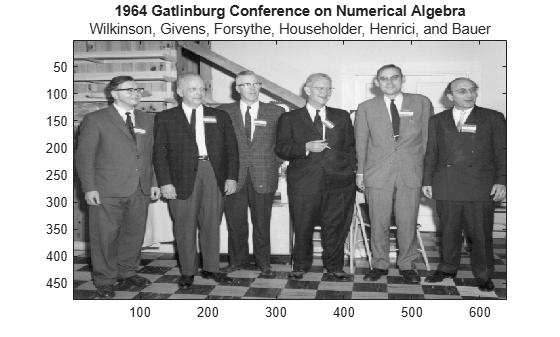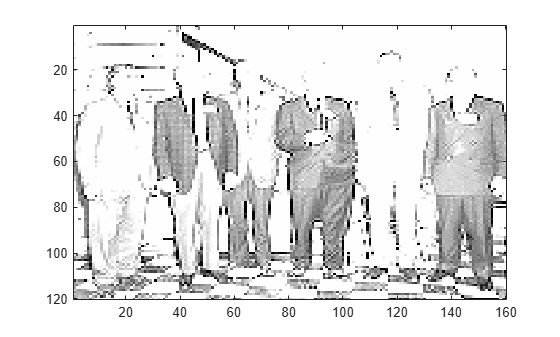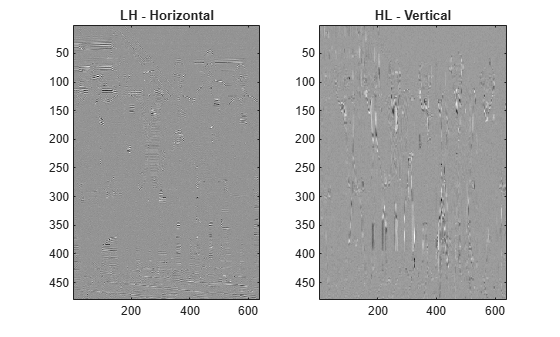# lwtcoef2

Extract 2-D LWT wavelet coefficients and orthogonal projections

## Syntax

``y = lwtcoef2(ll,lh,hl,hh)``
``y = lwtcoef2(ll,lh,hl,hh,Name=Value)``

## Description

````y = lwtcoef2(ll,lh,hl,hh)` returns the level 1 reconstructed approximation coefficients that correspond to the approximation coefficients `ll` and the horizontal (`lh`), vertical (`hl`), and diagonal (`hh`) wavelet coefficients. The coefficients in `ll`, `lh`, `hl`, and `hh` are the outputs of `lwt2` using default values.```

example

````y = lwtcoef2(ll,lh,hl,hh,Name=Value)` specifies options using one or more name-value arguments. For example, `lwtcoef2(ll,lh,hl,hh,Type="detail",OutputType="projection")` returns the projection of the detail coefficients at the finest scale using the `db1` wavelet.```

## Examples

collapse all

Load and plot a grayscale image.

```load gatlin figure image(X) colormap(map) title("1964 Gatlinburg Conference on Numerical Algebra",... "Wilkinson, Givens, Forsythe, Householder, Henrici, and Bauer")```Create a lifting scheme associated with the `bior3.7` wavelet. Use the lifting scheme to obtain the wavelet decomposition of the image to the maximum level.

```lscheme = liftingScheme(Wavelet="bior3.7"); [ll,lh,hl,hh] = lwt2(X,LiftingScheme=lscheme);```

Extract and display the approximation coefficients at level 2. Confirm the row and column dimensions are one-quarter the size of those of the original image.

```approxCF = lwtcoef2(ll,lh,hl,hh,... LiftingScheme=lscheme,OutputType="coefficients",Level=2); figure image(approxCF) colormap(map)````size(X)./size(approxCF)`
```ans = 1×2 4 4 ```

Obtain the orthogonal projection of the level 1 approximation coefficients. Also obtain the orthogonal projections of the detail coefficients at level 1. Display the projections corresponding to the `LH` and `HL` detail coefficients. Observe that the prominent features in the `LH`- and `HL`-derived images correspond to the horizontal, and vertical features, respectively, of the original image.

```approx = lwtcoef2(ll,lh,hl,hh,... LiftingScheme=lscheme,OutputType="projection",Level=1); dLH = lwtcoef2(ll,lh,hl,hh,... LiftingScheme=lscheme,OutputType="projection",Level=1,Type="LH"); dHL = lwtcoef2(ll,lh,hl,hh,... LiftingScheme=lscheme,OutputType="projection",Level=1,Type="HL"); dHH = lwtcoef2(ll,lh,hl,hh,... LiftingScheme=lscheme,OutputType="projection",Level=1,Type="HH"); subplot(1,2,1) imagesc(dLH) title("LH - Horizontal") subplot(1,2,2) imagesc(dHL) title("HL - Vertical")```Confirm the sum of the four projections equals the original image.

`max(max(abs(X-(approx+dLH+dHL+dHH))))`
```ans = 2.3448e-13 ```

## Input Arguments

collapse all

Approximation coefficients at the coarsest scale, specified as a scalar, vector, or matrix. The coefficients are the output of `lwt2`.

Data Types: `single` | `double`
Complex Number Support: Yes

Horizontal detail coefficients by level, specified as a LEV-by-1 cell array, where LEV is the level of the decomposition. The elements of `lh` are in order of decreasing resolution. The coefficients are the output of `lwt2`.

Data Types: `single` | `double`
Complex Number Support: Yes

Vertical detail coefficients by level, specified as a LEV-by-1 cell array, where LEV is the level of the decomposition. The elements of `hl` are in order of decreasing resolution. The coefficients are the output of `lwt2`.

Data Types: `single` | `double`
Complex Number Support: Yes

Diagonal detail coefficients by level, specified as a LEV-by-1 cell array, where LEV is the level of the decomposition. The elements of `hh` are in order of decreasing resolution. The coefficients are the output of `lwt2`.

Data Types: `single` | `double`
Complex Number Support: Yes

### Name-Value Arguments

Specify optional pairs of arguments as `Name1=Value1,...,NameN=ValueN`, where `Name` is the argument name and `Value` is the corresponding value. Name-value arguments must appear after other arguments, but the order of the pairs does not matter.

Example: ```y = lwtcoef2(ll,lh,hl,hh,OutputType="projection",LiftingScheme=lscheme)```

Orthogonal or biorthogonal wavelet, specified as a character vector or string scalar. See the Wavelet property of `liftingScheme` for the list of supported wavelets. The specified wavelet must match the value that you used to obtain the coefficients `ll`, `lh`, `hl`, and `hh`.

You cannot specify `Wavelet` and `LiftingScheme` at the same time.

Example: `y = lwtcoef2(ll,lh,hl,hh,Wavelet="bior3.5")` uses the `bior3.5` biorthogonal wavelet.

Data Types: `char` | `string`

Lifting scheme, specified as a `liftingScheme` object. The specified lifting scheme must be the same lifting scheme that you used to obtain the coefficients `ll`, `lh`, `hl`, and `hh`.

You cannot specify `LiftingScheme` and `Wavelet` at the same time.

Example: ```y = lwtcoef2(ll,lh,hl,hh,LiftingScheme=lScheme)``` uses the `lScheme` lifting scheme.

Output type, specified as one of these:

• `"coefficients"` — Extract the approximation or details coefficients

• `"projection"` — Return the projection (reconstruction) of the approximation or details coefficients

Example: ```y = lwtcoef2(ll,lh,hl,hh,OutputType="projection",Type="detail")``` returns the projection corresponding to the detail coefficients at the finest scale.

Type of coefficients to extract or reconstruct, specified as one of these:

• `"ll"` — Approximation coefficients

• `"lh"` — Horizontal coefficients

• `"hl"` — Vertical coefficients

• `"hh"` — Diagonal coefficients

Example: `y = lwtcoef2(ll,lh,hl,hh,Type="hh")` extracts the diagonal coefficients at the finest scale.

Level of coefficients to extract or reconstruct, specified as a positive integer less than or equal to `length(hh)`.

Example: ```y = lwtcoef2(ll,lh,hl,hh,LiftingScheme=lsc,Level=3)``` uses the lifting scheme `lsc` to extract the approximation coefficients at level 3.

Data Types: `double`

Extension mode to use to extract or reconstruct the coefficients, specified as one of these:

• `"periodic"` — Periodized extension

• `"zeropad"` — Zero extension

• `"symmetric"` — Symmetric extension

This argument specifies how to extend the signal at the boundaries. The extension mode must match the value you used to generate `ll`, `lh`, `hl`, and `hh`.

Example: ```y = lwtcoef2(ll,lh,hl,hh,Extension="zeropad")``` specifies zero extension.

Handling integer-valued data, specified as one of these:

• `1` (`true`) — Preserve integer-valued data

• `0` (`false`) — Do not preserve integer-valued data

`Int2Int` must match the value you used to generate `ll`, `lh`, `hl`, and `hh`.

Example: `y = lwtcoef2(ll,lh,hl,hh,Int2Int=true)` preserves integer-valued data.

## Output Arguments

collapse all

Extracted coefficients or projection, returns as a matrix. `y` has the same dimensionality as the input used by the `lwt2 ` function to generate the approximation and details coefficients.

Data Types: `single` | `double`

## Compatibility Considerations

expand all

Behavior changed in R2021b

## Extended Capabilities

### C/C++ Code GenerationGenerate C and C++ code using MATLAB® Coder™.